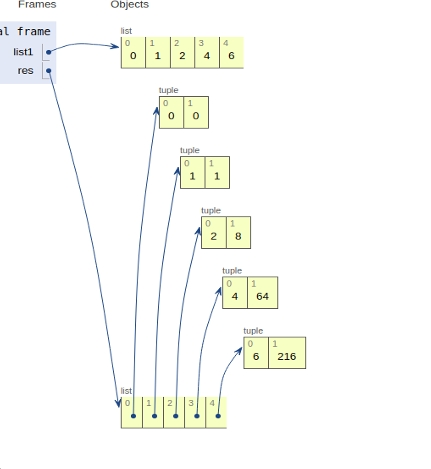# Python program to create a list of tuples from the given list having the number and its cube in each tuple

In this article, we will learn about the solution and approach to solve the given problem statement.

Problem statement −Given list input, we need to create a tuple with numbers and their corresponding cubes.

Let’s see the approach to solve the above problem with the help of inline implementation as shown.

## Example

Live Demo

list1 = [0,1,2,4,6]
res = [(val, pow(val, 3)) for val in list1]
# main
print(res)

## Output

[(0, 0), (1, 1), (2, 8), (4, 64), (6, 216)]

The figure given below shows the declaration of the list and its conversion into a series of nested tuples.## Conclusion

In this article, we learned about the approach to create a list of tuples from the given list having a number and its cube in each tuple.